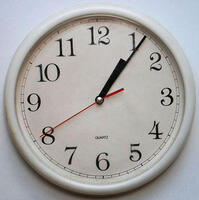Home > Patterns > Misunderstandings > Number lines > Drawing an analogue clock face

# Drawing an analogue clock face

The analogue clock face is a good example of a number line used as a measuring scale. It also provides a familiar context for investigating simple fractions.

Students can learn much about these and other ideas by investigating patterns on the clock face.

Here are some suggested learning activities. You can download the worksheet Draw Some Clocks.

• Ask students to draw a clock face in one of their circles. (Make sure there is no clock visible in the classroom!)
Discuss any difficulties they experience.
If students are unsure about the markings on a clock face, produce a real one or use the photo below.A clock with clearly labelled numerals.

• Cover up the clock again, and ask students to draw only the hour markings 3, 6, 9 and 12 on a new circle.
Students will need to understand that the numbers appear in 'clockwise' order.
Emphasise that these numbers divide the circle into four equal parts, and note the symmetry.
Explain the terms 'half past', 'quarter past' and 'quarter to' as fractions of an hour.
• Now fill in the missing hours by dividing each quarter segment into three equal parts. Students may need some practice at this.
• Finally, fill in the minutes. This time students must divide each space into five equal parts. Note that the circle has now been divided into 60 equal parts.